# Binary Heap Implementation

### Binary Heap:

A Binary Heap is a Binary Tree that satisfies the following conditions.

• It should be a Complete Binary Tree.
• It should satisfy the Heap property.

### Complete Binary Tree:

The tree in Which all the levels are completely filled except the last level and last level is filled in such a way that all the keys are as left as possible.

### Heap Property:

Binary Heap is either a Min Heap or Max Heap. Property of the Binary Heap decides whether it is Min Heap or Max Heap.

• Min Heap property: For every node in a binary heap, if the node value is less than its right and left child’s value then Binary Heap is known as Min Heap. The property of Node’s value less than its children’s value is known as Min Heap property. In Min Heap, the root value is always the Minimum value in Heap.
• Max Heap property: For every node in a binary heap, if the node value is greater than its right and left child’s value then Binary Heap is known as Max Heap. The property of being Node’s value greater than its children’s value is known as Max Heap property. In Max Heap, the root value is always the maximum value in Heap.

Representation of the Binary Heap:

A Binary Heap is represented as an array.

• The root value is at arr

The below table summarizes how the node and its children are stored in an array.

If the child is at index i, then its parent index can be found using the below formula.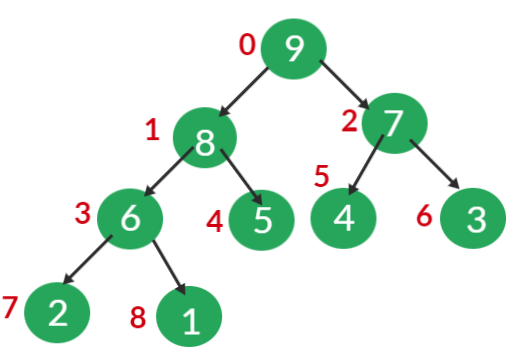Operations Associated with Min Heap:

• Insert()
• Heapify()
• getMin()
• ExtractMin()
• Delete()
• Decreasekey()

Let’s see how these operations are done Using Min Heap.

Note: The Binary heap should always be a complete binary tree and should satisfy the corresponding heap property (Min / Max). If any of the two conditions are disturbed we should make necessary modifications in a heap to satisfy the two conditions.

### Insert():

• Insert operation inserts a new key in the Binary Heap.

Steps Followed for inserting the key in Binary Heap:

• First Insert the key at the first vacant position from the left on the last level of the heap. IF the last level is completely filled, then insert the key as the left-most element in the next level.
• Inserting a new key at the first vacant position in the last level preserves the Complete binary tree property, The Min heap property may get affected we need to check for it.
• If the inserted key parent is less than the key, then the Min heap property is also not violated.
• If the parent is greater than the inserted key value, then swap the values. Now the heap property may get violated at the parent node. So repeat the same process until the heap property is satisfied.
• Inserting an element takes O(logN) Time Complexity. Below shows the animation of how -1 is inserted into a Binary heap by following above described steps.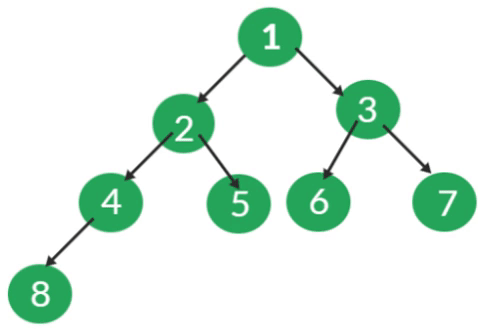### Heapify():

• Suppose there exists a Node at some index i, where the Minheap property is Violated.
• We assume that all the subtrees of the tree rooted at index i are valid binary heaps.
• The Heapify function is used to restore the Minheap, by fixing the violation.

Steps to be followed for Heapify:

• First find out the Minimum among the Violated Node, left, and right child of Violated Node.
• If the Minimum among them is the left child, then swap the Violated Node value with the Left child value and recursively call the function on the left Child.
• If the Minimum among them is the right child, then swap the Violated Node value with the right child value and recursively call the function right Child.
• Recursive call stops when the heap property is not violated.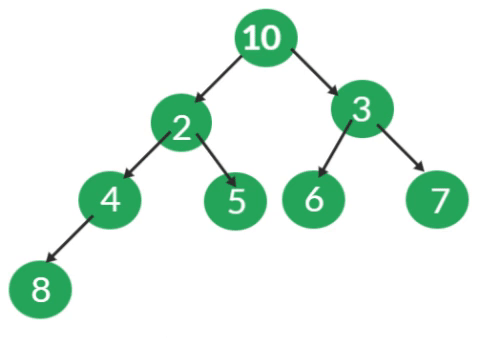### getMin():

• It returns the minimum value in the Binary Heap.
• As we all know, the root Node is the Minimum value in Min Heap. Simply return the arr.

### ExtractMin():

• This removes the Minimum element from the heap.

Steps to be followed to Remove Minimum value/root Node:

• Copy the last Node value to the Root Node, and decrease the size, this means that the root value is now deleted from the heap, and the size is decreased by 1.
• By doing the above step we ensure that the Complete binary tree property is not violated, as we are copying the last node value to the root node value, the Min Heap property gets violated.
• Call the Heapify function to convert it into a valid heap.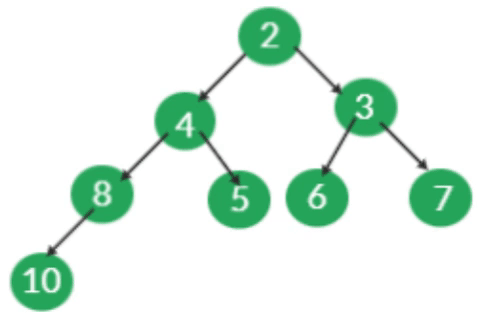### Decreasekey():

• Given an index and a value, we need to update the value at the index with the given value. We assume that the given value is less than the existing value at that index.

Steps to be followed for Decreasekey():

• Let’s the index be i and the value be new_val. Update existing value at index i with new_val i.e arr[i] = new_val.
• By performing the above step, the Complete binary tree property is not violated, but the Min heap property may get violated.
• As the new_val we are inserting is less than the previously existing value, the min-heap property is not violated in subtrees of this rooted tree.
• It may get violated in its ancestors, so as we do in insert operation, check the value of a current node with its parent node, if it violates the min-heap property

Swap the nodes and recursively do the same.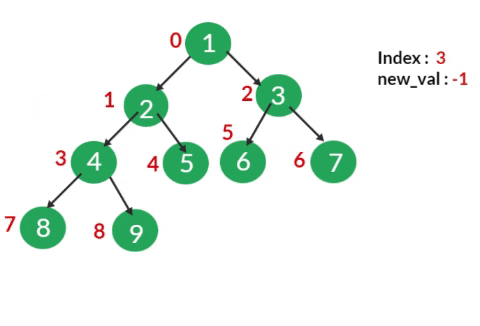### Delete() :

• Given an index, delete the value at that index from the min-heap.

Steps to be followed for Delete operation():

• First, update the value at the index that needs to be deleted with INT_MIN.
• Now call the Decreasekey() function at the index which is need to be deleted. As the value at the index is the least, it reaches the top.
• Now call the ExtractMin() operation which deletes the root node in Minheap.
• In this way, the desired index value is deleted from the Minheap.Code:

## C++ Code

``````#include <bits/stdc++.h>

using namespace std;

class BinaryHeap {
public:
int capacity; /*Maximum elements that can be stored in heap*/
int size; /*Current no of elements in heap*/
int * arr; /*array for storing the keys*/

BinaryHeap(int cap) {
capacity = cap; /*Assigning the capacity*/
size = 0; /*Intailly size of hepa is zero*/
arr = new int[capacity]; /*Creating a array*/
}

/*returns the parent of ith Node*/
int parent(int i) {
return (i - 1) / 2;
}
/*returns the left child of ith Node*/
int left(int i) {
return 2 * i + 1;
}
/*Returns the right child of the ith Node*/
int right(int i) {
return 2 * i + 2;
}

/*Insert a new key x*/
void Insert(int x) {
if (size == capacity) {
cout << "Binary Heap Overflwon" << endl;
return;
}
arr[size] = x; /*Insert new element at end*/
int k = size; /*store the index ,for checking heap property*/
size++; /*Increase the size*/

/*Fix the min heap property*/
while (k != 0 && arr[parent(k)] > arr[k]) {
swap( & arr[parent(k)], & arr[k]);
k = parent(k);
}
}

void Heapify(int ind) {
int ri = right(ind); /*right child*/
int li = left(ind); /*left child*/
int smallest = ind; /*intially assume violated value in Min value*/
if (li < size && arr[li] < arr[smallest])
smallest = li;
if (ri < size && arr[ri] < arr[smallest])
smallest = ri;
/*smallest will store the minvalue index*/
/*If the Minimum among the three nodes is the parent itself,
that is Heap property satisfied so stop else call function recursively on Minvalue node*/
if (smallest != ind) {
swap( & arr[ind], & arr[smallest]);
Heapify(smallest);
}
}
int getMin() {
return arr;
}
int ExtractMin() {
if (size <= 0)
return INT_MAX;
if (size == 1) {
size--;
return arr;
}
int mini = arr;
arr = arr[size - 1]; /*Copy last Node value to root Node value*/
size--;
Heapify(0); /*Call heapify on root node*/
return mini;
}
void Decreasekey(int i, int val) {
arr[i] = val; /*Updating the new_val*/
while (i != 0 && arr[parent(i)] > arr[i]) /*Fixing the Min heap*/ {
swap( & arr[parent(i)], & arr[i]);
i = parent(i);
}
}
void Delete(int i) {
Decreasekey(i, INT_MIN);
ExtractMin();
}
void swap(int * x, int * y) {
int temp = * x;
* x = * y;
* y = temp;
}
void print() {
for (int i = 0; i < size; i++)
cout << arr[i] << " ";
cout << endl;
}
};
int main()

{
BinaryHeap h(20);
h.Insert(4);
h.Insert(1);
h.Insert(2);
h.Insert(6);
h.Insert(7);
h.Insert(3);
h.Insert(8);
h.Insert(5);
cout << "Min value is " << h.getMin() << endl;
h.Insert(-1);
cout << "Min value is " << h.getMin() << endl;
h.Decreasekey(3, -2);
cout << "Min value is " << h.getMin() << endl;
h.ExtractMin();
cout << "Min value is " << h.getMin() << endl;
h.Delete(0);
cout << "Min value is " << h.getMin() << endl;
return 0;
}``````

Output:

Min value is 1
Min value is -1
Min value is -2
Min value is -1
Min value is 1

## Java Code

``````import java.util.*;

class BinaryHeap {
static int capacity; /*Maximum elements that can be stored in heap*/
static int size; /*Current no of elements in heap*/
static int arr[]; /*array for storing the keys*/

BinaryHeap(int cap) {
capacity = cap; /*Assigning the capacity*/
size = 0; /*Intailly size of hepa is zero*/
arr = new int[capacity]; /*Creating a array*/
}

/*returns the parent of ith Node*/
static int parent(int i) {
return (i - 1) / 2;
}
/*returns the left child of ith Node*/
static int left(int i) {
return 2 * i + 1;
}
/*Returns the right child of the ith Node*/
static int right(int i) {
return 2 * i + 2;
}

/*Insert a new key x*/
static void Insert(int x) {
if (size == capacity) {
System.out.println("Binary Heap Overflown");
return;
}
arr[size] = x; /*Insert new element at end*/
int k = size; /*store the index ,for checking heap property*/
size++; /*Increase the size*/

/*Fix the min heap property*/
while (k != 0 && arr[parent(k)] > arr[k]) {
int temp = arr[parent(k)];
arr[parent(k)] = arr[k];
arr[k] = temp;
k = parent(k);
}
}

static void Heapify(int ind) {
int ri = right(ind); /*right child*/
int li = left(ind); /*left child*/
int smallest = ind; /*intially assume violated value in Min value*/
if (li < size && arr[li] < arr[smallest])
smallest = li;
if (ri < size && arr[ri] < arr[smallest])
smallest = ri;
/*smallest will store the minvalue index*/
/*If the Minimum among the three nodes is the parent itself,
that is Heap property satisfied so stop else call function recursively on Minvalue node*/
if (smallest != ind) {
int temp = arr[ind];
arr[ind] = arr[smallest];
arr[smallest] = temp;
Heapify(smallest);
}
}
static int getMin() {
return arr;
}
static int ExtractMin() {
if (size <= 0)
return Integer.MAX_VALUE;
if (size == 1) {
size--;
return arr;
}
int mini = arr;
arr = arr[size - 1]; /*Copy last Node value to root Node value*/
size--;
Heapify(0); /*Call heapify on root node*/
return mini;
}
static void Decreasekey(int i, int val) {
arr[i] = val; /*Updating the new_val*/
while (i != 0 && arr[parent(i)] > arr[i]) /*Fixing the Min heap*/ {
int temp = arr[parent(i)];
arr[parent(i)] = arr[i];
arr[i] = temp;
i = parent(i);
}
}
static void Delete(int i) {
Decreasekey(i, Integer.MIN_VALUE);
ExtractMin();
}
static void print() {
for (int i = 0; i < size; i++)
System.out.print(arr[i] + " ");
System.out.println();
}
public static void main(String args[])

{
BinaryHeap h = new BinaryHeap(20);
h.Insert(4);
h.Insert(1);
h.Insert(2);
h.Insert(6);
h.Insert(7);
h.Insert(3);
h.Insert(8);
h.Insert(5);
System.out.println("Min value is " + h.getMin());
h.Insert(-1);
System.out.println("Min value is " + h.getMin());
h.Decreasekey(3, -2);
System.out.println("Min value is " + h.getMin());
h.ExtractMin();
System.out.println("Min value is " + h.getMin());
h.Delete(0);
System.out.println("Min value is " + h.getMin());

}
}``````

Output:

Min value is 1
Min value is -1
Min value is -2
Min value is -1
Min value is 1